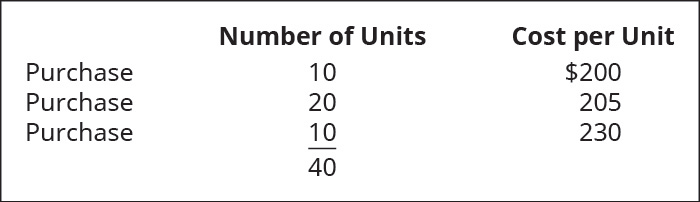# When prices are falling (deflation), which costing method would produce the highest gross margin for the following? Choose first-in, first-out (FIFO); last-in, first-out (LIFO); or weighted average, assuming that B62 Company had the following transactions for the month. Calculate the gross margin for each of the following cost allocation methods, assuming B62 sold just one unit of these goods for \$400. Provide your calculations. A. first-in, first-out (FIFO) B. last-in, first-out (LIFO) C. weighted average (AVG)FindFindarrow_forward

### Principles of Accounting Volume 1

19th Edition
OpenStax
Publisher: OpenStax College
ISBN: 9781947172685

#### Solutions

Chapter
SectionFindFindarrow_forward

### Principles of Accounting Volume 1

19th Edition
OpenStax
Publisher: OpenStax College
ISBN: 9781947172685
Chapter 10, Problem 1PB
Textbook Problem
9 views

## When prices are falling (deflation), which costing method would produce the highest gross margin for the following? Choose first-in, first-out (FIFO); last-in, first-out (LIFO); or weighted average, assuming that B62 Company had the following transactions for the month.Calculate the gross margin for each of the following cost allocation methods, assuming B62 sold just one unit of these goods for \$400. Provide your calculations.A. first-in, first-out (FIFO)B. last-in, first-out (LIFO)C. weighted average (AVG)

To determine

Concept introduction:

FIFO (First in first out): In FIFO Method Company issues first that material which is purchased earliest. This method provide highest gross margin in case of inflation.

LIFO (Last in first out): In LIFO Method Company issues first that material which comes in last in inventory. This method provide least gross margin in case of inflation.

Weighted average method: In this method we issue material on random basis, so every type of goods has equal probability.

Requirement-1:

To calculate:

Gross margin under FIFO.

### Explanation of Solution

Gross margin as per FIFO= Sale value − Co...

To determine

Concept introduction:

FIFO (First in first out): In FIFO Method Company issues first that material which is purchased earliest. This method provide highest gross margin in case of inflation.

LIFO (Last in first out): In LIFO Method Company issues first that material which comes in last in inventory. This method provide least gross margin in case of inflation.

Weighted average method: In this method we issue material on random basis, so every type of goods has equal probability.

Requirement-2:

To calculate:

Gross margin under LIFO.

To determine

Concept introduction:

FIFO (First in first out): In FIFO Method Company issues first that material which is purchased earliest. This method provide highest gross margin in case of inflation.

LIFO (Last in first out): In LIFO Method Company issues first that material which comes in last in inventory. This method provide least gross margin in case of inflation.

Weighted average method: In this method we issue material on random basis, so every type of goods has equal probability.

Requirement-3:

To calculate:

Gross margin under Weighted Average Method.

### Still sussing out bartleby?

Check out a sample textbook solution.

See a sample solution

#### The Solution to Your Study Problems

Bartleby provides explanations to thousands of textbook problems written by our experts, many with advanced degrees!

Get Started

Find more solutions based on key concepts
What is the bullwhip effect?

Accounting Information Systems

DIVIDEND POLICY Southeastern Steel Company (SSC) was formed 5 years ago to exploit a new continuous casting pro...

Fundamentals of Financial Management, Concise Edition (with Thomson ONE - Business School Edition, 1 term (6 months) Printed Access Card) (MindTap Course List)

Why should policymakers care about GDP?

Principles of Macroeconomics (MindTap Course List)